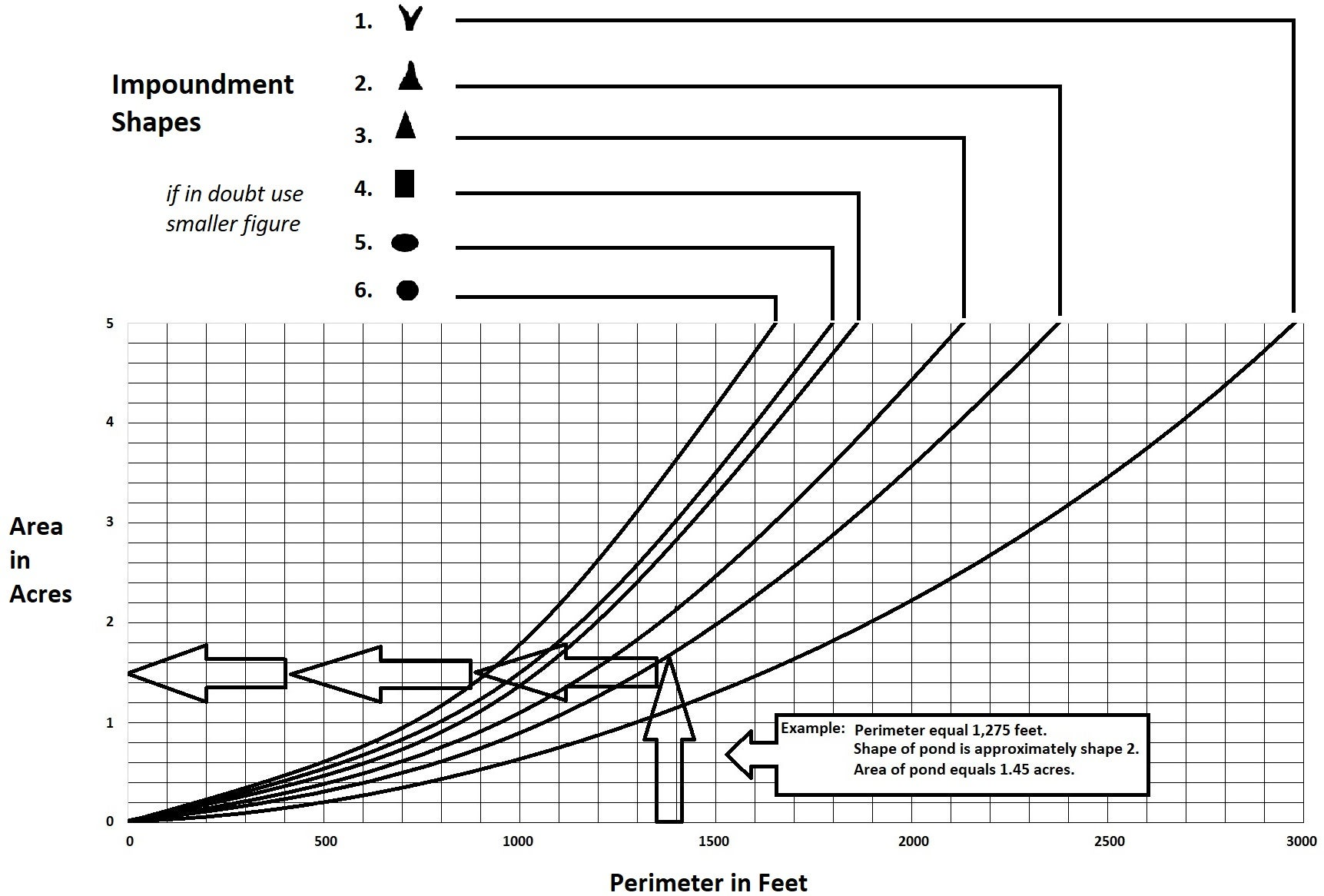# Pond Area Estimator

Body

## Determine the Number of Acre-Feet in Your Pond to Calculate the Amount of Herbicide Needed

If the acreage of the area to be treated is known, the number of acre-feet can be determined by multiplying the number of acres by the average depth (average depth = 1/3 of the maximum depth). For example: A two acre area is to be treated and has an average depth of three feet. The volume of the water is six acre-feet.

2 acres x 3 feet (average depth) = 6 acre-feet

If the dosage of herbicide recommended is 2 gallons of herbicide per acre-foot, the total herbicide needed would be twelve gallons.

6 acre-feet x 2 gallon/acre-foot = 12 gallons (total herbicide needed)

If the number of acres is not known, it can be estimated by measuring the number of square feet and dividing by 43,560. The number of square feet in many cases can be closely approximated by multiplying the average width in feet by the average length in feet. For example: A shoreline area is to be treated. The weeded area is 500 feet long and averages 10 feet wide. The total surface area is 5,000 square feet or 0.115 acres.

10 feet x 500 feet = 5,000 square feet
5,000 square feet ∙ 43,560 (square feet in an acre) = 0.115 acres

The average depth of water in this shoreline area is 1 foot. The total acre-feet is 0.115.

0.115 acres x 1 foot (average depth) = 0.115 acre-feet

If we assume that 4 gallon/acre-foot was the recommended dosage, then 0.46 gallons of herbicide would be needed.

4 gallon/acre-foot x 0.115 (acre feet) = 0.46 gallons (total herbicide needed)

Body